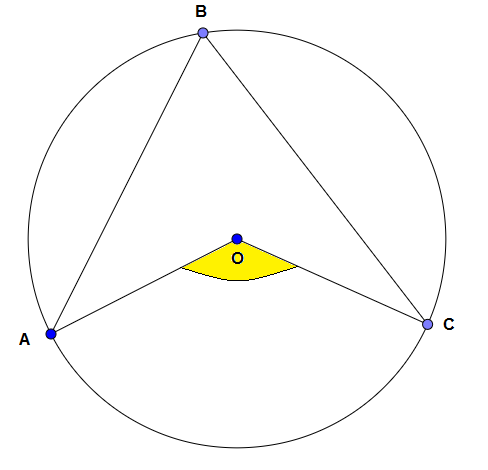# Almost A Central Angle

Geometry Level 2In the figure above, $O$ is the center of the circle, $\angle BAO = 30^\circ$ and $\angle BCO = 40^\circ$. Find the shaded angle $\angle AOC$.

×

Problem Loading...

Note Loading...

Set Loading...